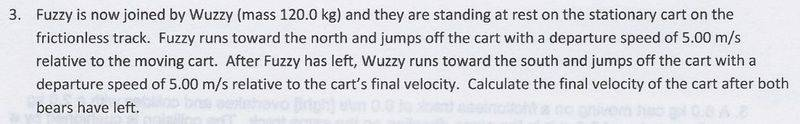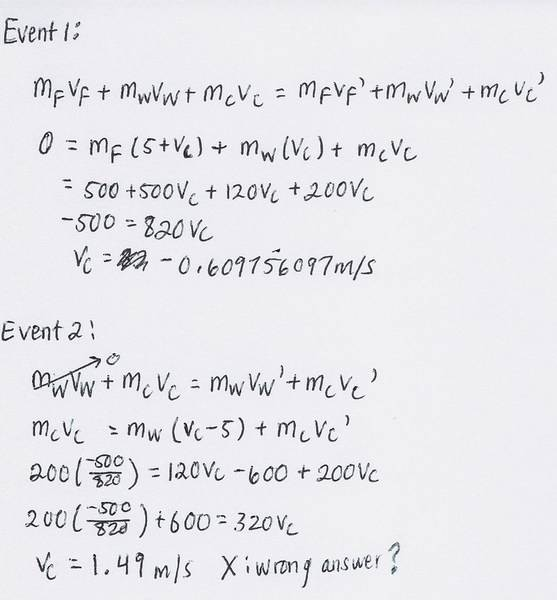# Conservation of Momentum and Relative Velocity Problem

• whoareyou
In summary, the homework statement is that two masses, one of which is much larger than the other, will split into two smaller masses when they collide. Conservation of momentum is applied twice to determine the velocities of the two masses after the collision.f

## Homework Statementmass of Fuzzy = 100.0kg
mass of cart = 200.0kg

## Homework Equations

Conservation of Momentum for 1D Motion

Relative Velocity for 1D Motion

## The Attempt at a Solution

Since everything is at rest at the beginning, the total momentum of the system is 0. So at the end, 0 = m1v1 + m2v2 --> m1v1 = -m2v2, so m1v1 = -m(5+v1), and after expanding that, and solving for v1, the answer isn't correct. The correct answer is 0.685m/s [N]. IS my approach wrong? I think it might be do to a change in the mass of the system, but even if that is the right way to go, I don't know how to incorporate that in the equation.

In this problem, there are two "events" in which a larger mass splits up into two smaller ones. In introductory physics, these events are often described as "explosions" as a sort of opposite to "collisions". But whatever name you choose to give to the events, it is helpful to apply conservation of momentum TWICE. Once for each "splitting" event.

Event 1: Conservation of momentum

momentum before = momentum after

where "before" means before Fuzzy jumped off the cart and "after" means after Fuzzy jumped off the cart.

Use conservation of momentum in this situation to determine the velocity of the cart after Fuzzy jumps off. Then apply that velocity as an initial condition to event 2:

Event 2: Conservation of Momentum

momentum before = momentum after

where "before" means before Wuzzy jumped off the cart, and "after" means after Wuzzy jumped off the cart.

k I did my work, but I still got the wrong answer. I'll scan my work.

I still end up with the wrong answer. The correct answer is 0.685m/s.You're complicating this TOO much. Initially there is ONE object of mass Mtot = mw + mf + mc

After event 1, there are TWO objects, one of mass mf, and one of mass M = mw + mc. So, conservation of momentum says:

0 = mfvf + Mv

where v is what I'm calling the velocity of (cart + Wuzzy) after Fuzzy jumps off.

mfvf = -Mv

Now, let's arbitrarily choose North as the positive direction. We are told that Fuzzy moves at speed +5 m/s relative to M, or in other words,

vf - v = 5 m/s

vf = (5 m/s) + v

substituting, we get:

(5 m/s)mf + mfv = -Mv

mfv +Mv = -(5 m/s)mf

(mf + M)v = -(5 m/s)mf

v = - [ (5 m/s)mf ] / [mf + M]

DON'T PLUG IN ANY NUMBERS YET. What would be the point? It would just introduce needless intermediate arithmetic steps. Keep things algebraic until you have the expression that will allow you compute the final answer.

For event 2, we initially have one object of mass M moving with velocity v. After the collision, we have two objects of mass mc and mw. Conservation of momentum says that:

Mv = mwvw + mcvc

Now, we are told that Wuzzy is moving at a velocity of -5 m/s relative to the cart. In other words:

vw - vc = -5 m/s

vw = vc - 5 m/s

Substituting this into the consv. of momentum equation:

Mv = mw(vc - 5 m/s) + mcvc

Mv = mwvc -mw(5m/s) + mcvc

Mv + mw(5m/s) = vc(mw + mc)

vc = [Mv + mw(5 m/s)] / (mw + mc)

Now, substituting in the expression for v, this becomes:

$$v_c = \frac{(-5m_fM)/(m_f + M) + 5m_w}{m_w + m_c}$$

NOW plug in the values. If I do this, keeping in mind that M = mc + mw, I get +0.6845 m/s.

I encourage you to scrutinize every step of this solution, and if there's something you don't understand, ask me about it. The physics of the problem is easy. It is merely algebraically messy.

After reading only the first two lines, I redid it and got the right answer! Thanks for your help!